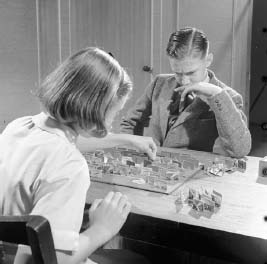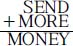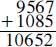NextPrevious

# What is a cryptarithmetic puzzle?

Some of the most challenging verbal arithmetic puzzles are called cryptarithmetic puzzles. These number puzzles (often called cryptarithms) are made up of mathematical equations whose digits are represented by letters or symbols; the goal is to identify the numerical value of each letter. In such a puzzle, each letter represents a unique digit, but there are rules. As in ordinary arithmetic notation, the leading digit of a multi-digit number must not be zero; also, the puzzle usually has only one solution.Playing games with puzzles that often demand mathematical skills has been a popular pastime for generations.

Cryptarithmetic puzzles are most often divided into two types. An alphametic cryptarithm is one in which the letters are used to represent distinct digits. These are derived from related words or meaningful phrases in the puzzles. A digimetic cryptarithm is one in which the digits are used to represent other digits. One of the most famous cryptarithmetic puzzles was developed by Englishman Henry Ernest Dudeney (1857–1930) and published in a 1924 issue of the Strand Magazine:In this puzzle (developed by Englishman Henry Ernest Dudeney [1857-1930] and published in 1924 in the Strand Magazine), the addition the addition sum of each letter represents a digit, with all the letters being different digits. These letters represent the numbers as follows: O = 0, M = 1, Y = 2, E = 5, N = 6, D = 7, R = 8, and S = 9. OrClose

This is a web preview of the "The Handy Math Answer Book" app. Many features only work on your mobile device. If you like what you see, we hope you will consider buying. Get the App